﻿ 均匀圆形阵列天线的压缩感知二维DOA估计方法

# 均匀圆形阵列天线的压缩感知二维DOA估计方法Compressed Sensing 2-D DOA Estimation for the Uniform Circular Array

Abstract: Combining the particularity of the uniform circular array structure, this paper proposes a 2-D DOA estimation method based on CS theory. This method first uses the pattern space transformation to convert the uniform circular array of array element space into the virtual linear array of pattern space; secondly, the SVD processing is used to reduce the dimension to obtain a low-dimensional data matrix; finally, utilizes the OMP algorithm to solve the problem to obtain the 2-D angle estima-tion value. Theoretical derivation and experimental simulations show that this method does not re-quire spectral peak search and has a small amount of calculation. It also has good estimation per-formance for two-dimensional coherent and non-coherent signals. Compared with the classic algo-rithm, this method also has certain advantages for 2-D DOA estimation under different conditions.

1. 引言

2. 压缩感知理论Figure 1. Compressed sensing process

$x=\underset{i=1}{\overset{N}{\sum }}{\Psi }_{i}{S}_{i}=\Psi S$ (1)

$y=\Phi x$ (2)

$y=\Phi x=\Phi \Psi S=\Theta S$ (3)

$\mathrm{min}{‖S‖}_{0}\text{\hspace{0.17em}}\text{\hspace{0.17em}}\text{s}\text{.t}.\text{\hspace{0.17em}}\text{\hspace{0.17em}}y=\Theta S$ (4)

$\mathrm{min}{‖S‖}_{1}\text{\hspace{0.17em}}\text{\hspace{0.17em}}\text{s}\text{.t}\text{.}\text{\hspace{0.17em}}\text{\hspace{0.17em}}y=\Theta S$ (5)

$y=\Phi x+n=\Phi \Psi S+n=\Theta S+n$ (6)

$\mathrm{min}{‖S‖}_{1}\text{\hspace{0.17em}}\text{\hspace{0.17em}}\text{\hspace{0.17em}}\text{s}\text{.t}.\text{\hspace{0.17em}}\text{\hspace{0.17em}}{‖y-\Theta S‖}_{2}<\epsilon$ (7)

3. 基于压缩感知的二维DOA估计

3.1. 阵列结构和信号模型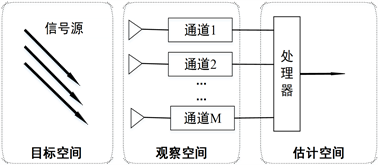Figure 2. The spatial spectrum estimation process

1) 目标信号为远场窄带信号，阵列接收到的信号可视为平面波；

2) 天线阵列为全向天线，入射平面波的波长远，接收阵列阵元间距大于其几何尺寸，且各个阵元间的相互影响可忽略不计；

3) 信号在信道中的传输介质均匀、线性、同向性及非扩散的。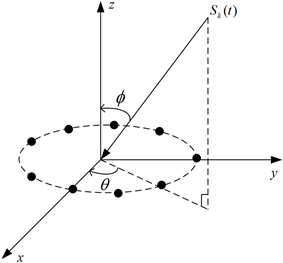Figure 3. The uniform circular array structure

${y}_{m}\left(t\right)=\underset{k=1}{\overset{K}{\sum }}a\left({\theta }_{k},{\varphi }_{k}\right){s}_{k}\left(t\right)+{n}_{m}\left(t\right)$ (8)

$a\left({\theta }_{k},{\varphi }_{k}\right)={\left[{\text{e}}^{j2\pi r\mathrm{sin}{\varphi }_{k}\mathrm{cos}\left({\theta }_{k}\right)/\lambda },{\text{e}}^{j2\pi r\mathrm{sin}{\varphi }_{k}\mathrm{cos}\left({\theta }_{k}-\frac{2\pi }{M}\right)/\lambda },\cdots ,{\text{e}}^{j2\pi r\mathrm{sin}{\varphi }_{k}\mathrm{cos}\left({\theta }_{k}-\frac{2\pi \left(M-1\right)}{M}\right)/\lambda }\right]}^{\text{T}}$ (9)

$y\left(t\right)=A\left(\theta ,\varphi \right)s\left(t\right)+n\left(t\right)$ (10)

$a\left({\theta }_{k},{\varphi }_{k}\right)={\left[{\text{e}}^{j\varsigma \mathrm{cos}\left({\theta }_{k}-{\gamma }_{0}\right)},{\text{e}}^{j\varsigma \mathrm{cos}\left({\theta }_{k}-{\gamma }_{1}\right)},\cdots ,{\text{e}}^{j\varsigma \mathrm{cos}\left({\theta }_{k}-{\gamma }_{M-1}\right)}\right]}^{\text{T}}$ (11)

$Y=A\left(\theta ,\varphi \right)S+N$ (12)

3.2. 模式空间转换

$\begin{array}{l}F=W/\sqrt{M}\\ W={\left[{w}_{-h},{w}_{-h+1},\cdots ,{w}_{0},\cdots ,{w}_{h-1},{w}_{h}\right]}^{\text{T}}\\ {w}_{i}=\left[1,{\text{e}}^{ji{\gamma }_{0}},\cdots ,{\text{e}}^{ji{\gamma }_{M-1}}\right]\end{array}$ (13)

${w}_{i}^{\text{H}}a\left({\theta }_{k},{\varphi }_{k}\right)={b}_{i}\left({\varphi }_{k}\right){\text{e}}^{ji{\theta }_{k}}+\underset{q=1}{\overset{\text{+}\infty }{\sum }}\left[{b}_{i+qM}\left({\varphi }_{k}\right){\text{e}}^{j\left(i+qM\right){\theta }_{k}}+{b}_{i-qM}\left({\varphi }_{k}\right){\text{e}}^{j\left(i-qM\right){\theta }_{k}}\right]$ (14)

${w}_{i}^{\text{H}}a\left({\theta }_{k},{\varphi }_{k}\right)\approx {b}_{i}\left({\varphi }_{k}\right){\text{e}}^{ji{\theta }_{k}}$ (15)

${b}_{i}^{-1}\left({\varphi }_{k}\right){w}_{i}^{\text{H}}a\left({\theta }_{k},{\varphi }_{k}\right)\approx {\text{e}}^{ji{\theta }_{k}}$ (16)

$\stackrel{¯}{Y}=JFY=JFA\left(\theta ,\varphi \right)S+JFN=\stackrel{¯}{A}\left(\theta \right)S+JFN$ (17)

$\begin{array}{l}J=diag\left[1/{b}_{i}\left(\theta \right)\right]\\ \stackrel{¯}{A}\left(\theta \right)=\left[\begin{array}{cccc}{\text{e}}^{-jh{\theta }_{1}}& {\text{e}}^{-jh{\theta }_{2}}& \cdots & {\text{e}}^{-jh{\theta }_{K}}\\ {\text{e}}^{-j\left(h-1\right){\theta }_{1}}& {\text{e}}^{-j\left(h-1\right){\theta }_{2}}& \cdots & {\text{e}}^{-j\left(h-1\right){\theta }_{K}}\\ ⋮& ⋮& \ddots & ⋮\\ {\text{e}}^{jh{\theta }_{1}}& {\text{e}}^{jh{\theta }_{2}}& \cdots & {\text{e}}^{jh{\theta }_{K}}\end{array}\right]\end{array}$ (18)

3.3. SVD处理方法

$\stackrel{¯}{Y}=UL{V}^{\text{H}}$ (19)

${\stackrel{¯}{Y}}_{\text{S}}=UL{D}_{\text{k}}=\stackrel{¯}{Y}V{D}_{\text{k}}$ (20)

3.4. 稀疏信号求解Table 1. 2-D DOA estimation algorithm steps

4. 实验仿真与分析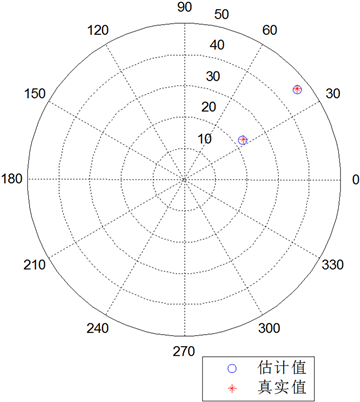Figure 4. Two-dimensional DOA estimation of two signal sources

$p=\frac{{N}_{suc}}{{N}_{trial}}×100\text{%}$ (21)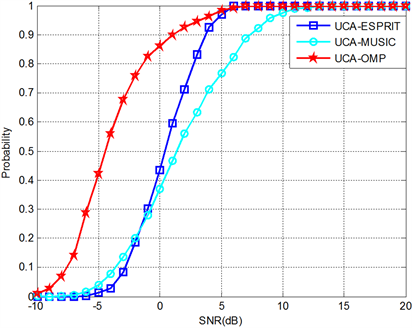Figure 5. Comparison of the success probabilities of several algorithms under different SNR

$\begin{array}{l}RMSE=\frac{1}{K}\underset{k=1}{\overset{K}{\sum }}\sqrt{\frac{1}{N}\underset{n=1}{\overset{N}{\sum }}{\left({\stackrel{^}{\theta }}_{kn}-{\theta }_{k}\right)}^{2}}\\ RMSE=\frac{1}{K}\underset{k=1}{\overset{K}{\sum }}\sqrt{\frac{1}{N}\underset{n=1}{\overset{N}{\sum }}{\left({\stackrel{^}{\varphi }}_{kn}-{\varphi }_{k}\right)}^{2}}\end{array}$ (22)

$RMSE=\frac{1}{K}\underset{k=1}{\overset{K}{\sum }}\sqrt{\frac{1}{N}\underset{n=1}{\overset{N}{\sum }}\left({\left({\stackrel{^}{\theta }}_{kn}-{\theta }_{k}\right)}^{2}\text{+}{\left({\stackrel{^}{\varphi }}_{kn}-{\varphi }_{k}\right)}^{2}\right)}$ (23)

5. 结论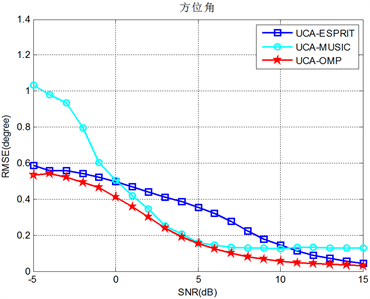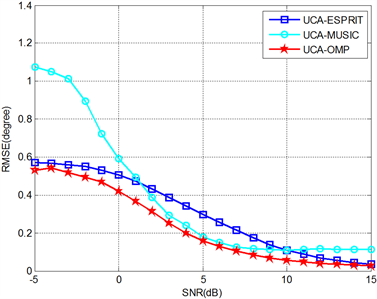Figure 6. The RMSE comparison of several algorithms under different SNR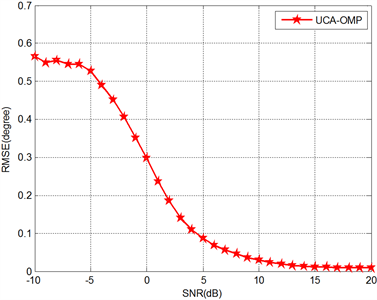Figure 7. The RMSE-SNR plot of the algorithm under coherent sources

1) 无需空间平滑等预处理即可实现相干信源的DOA估计；

2) 利用模式转换，使得阵列流型矩阵满足范德蒙矩阵要求；

3) 通过奇异值分解将阵列接收数据进行降维，得到低维的信号子空间，降低了运算的复杂度，提高了算法的抗噪性能。

NOTES

*通讯作者。

 王永良, 陈辉, 彭应宁, 万群. 空间谱估计理论与算法[M]. 北京: 清华大学出版社, 2004.

 Wang, L. and Fei, W. (2012) A Joint Estimation Method of Azimuth, Elevation, Range and Polarization for Near Field Sources Based on Second Order Statistics. Proceedings of 2011 International Conference on Computer Science and Network Technology, Harbin, 24-26 December 2011, 771-775.

 Fu, T., Chen, J. and Gao, X. (2006) Joint Angle and Frequency Estima-tion with Uniform Eigenvalue Weighting. First International Conference on Communications and Networking in China, Beijing, 25-27 October 2006, 25-27.
https://doi.org/10.1109/CHINACOM.2006.344657

 Chen, Y.F. and Yen, S.L. (2002) Smart Antenna with Joint Angle and Delay Estimation for the Geolocation,SMART Uplink and Downlink Beamforming. 6th International Conference on Signal Processing, Beijing, 26-30 August 2002, 393-397.

 Tohanean, C. and Picheral, J. (2007) BER Performance Improvement with Joint Angle-Delay-Polarization Estimation of Multipath Channel Parameters. IEEE Vehicular Technology Conference, Montreal, 25-28 September 2006, 1-5.
https://doi.org/10.1109/VTCF.2006.31

 Hu, Z.H., He, F. and Zhu, J.B. (2011) L-Shaped 2-D DOA Estimation Using Blind Source Separation. Proceedings of SPIE: The International Society for Optical Engineering, Cairo, Egypt, 1-2 October 2011, 8285.

 Mathews, C.P. and Zoltowski, M.D. (1994) Eigenstructure Techniques for 2-D Angle Estimation with Uniform Circular Arrays. IEEE Transactions on Signal Processing, 42, 2395-2407.
https://doi.org/10.1109/78.317861

 Askari, M. and Karimi, M. (2013) Sector Beamforming with Uniform Circular Array Antennas Using Phase Mode Transformation. 21st Iranian Conference on Electrical Engineering, Mashhad, 14-16 May 2013, 1-6.
https://doi.org/10.1109/IranianCEE.2013.6599708

 潘捷, 周建江, 汪飞. 非均匀噪声稀疏均匀圆阵的二维DOA估计[J]. 航空学报, 2011(3): 75-83.

 Roos, F. et al. (2019) Compressed Sensing Based Single Snapshot DoA Estimation for Sparse MIMO Radar Arrays. 12th German Microwave Conference, Stuttgart, 75-78.
https://doi.org/10.23919/GEMIC.2019.8698136

 Xue, H.X. and Zhao, Y.J. (2013) DOA Estimation Based on Compressive Sampling Array. Journal of Electronic Measurement & Instrument, 26, 208-214.
https://doi.org/10.3724/SP.J.1187.2012.00208

 Du, Z.-M., Ye, F.-Y., Shi, H., et al. (2015) A Fast Recovery Method of 2D Geometric Compressed Sensing Signal. Circuits Systems & Signal Processing, 34, 1711-1724.
https://doi.org/10.1007/s00034-014-9913-3

 Candes, E.J., Romberg, J. and Tao, T. (2004) Robust Uncertainty Principles: Exact Signal Reconstruction from Highly Incomplete Frequency Information. IEEE Transactions on In-formation Theory, 52, 489-509.
https://doi.org/10.1109/TIT.2005.862083

 Asif, M.S. and Romberg, J. (2012) Fast and Accurate Algorithms for Re-Weighted L1-Norm Minimization. IEEE Transactions on Signal Processing, 61, 5905-5916.
https://doi.org/10.1109/TSP.2013.2279362

 Malioutov, D.M., Müjdat, Ç. and Willsky, A.S. (2005) Sparse Signal Reconstruction Perspective for Source Localization with Sensor Arrays. IEEE Transactions on Signal Processing, 53, 3010-3022.
https://doi.org/10.1109/TSP.2005.850882

 Xu, Z. (2012) The Performance of Orthogonal Multi-Matching Pursuit under RIP.

Top